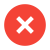﻿ Corrosionrate - MPY - Mils per year - 1 mil in mm

# Mils per Year – MPY – Corrosion Rate

Mils per year or MPY is used to give the corrosion rate in a pipe, a pipe system or other metallic surfaces. It is used to calculate the material loss or weight loss of a metal surfaces. There is a formula using the type of metal, the size of the sample area and the time of exposure, giving the value of mils per year.

# Convert 1 mil to mm

equals ```function mpycalc() {var v_um,eingabe;v_um=document.getElementById("mpyeingabe").value.replace(",",".")*document.getElementById("mpyauswahl").value ;document.getElementById("mpyausgabe").value=Math.round(1000*v_um/0.0254)/1000 + " mil";document.getElementById("mmpyausgabe").value=Math.round(1000*v_um)/1000 + " mm" ; ga('send', 'event', 'umrechnung', 'mil-mm'); }```

The expression MPY is mostly used in the United States. To convert mils into inches, you just have to add or remove 3 digits. 1 Mils is one thousandth of an Inch or 0.001 Inch. And the other way around 1 Inch is 1000 mils. In metric expression one mil equals to 0.0254 mm. The corrosion rate can be also calculated in MMY, means Millimeter per year.

In an open water system a corrosion rate of around 1 MPY is normal. Having corrosion rate of around 10,  you should take action. Corrosion rates of 20 MPY and above, you should be concerned, as the corrosion is „eating“ the metal rather fast.

### MPY is a rather dry figure, here an example

A 24″ pipeline and a corrosion rate of 10 MPY, will result in almost half a cubic meter material loss per kilometer pipe length. Or app. 20 cubic feet per 0.6 miles. Such amount of rust gives big problems on the receiver side at the end of the pipe line. This figure is most important to calculate the life time of a pipe. E.g in a gas pipe line, where the pressure is rather high, it is very critical to know how long the pipe material will last and the pipe can be used.

Having pitting corrosion in a pipe, there is sometimes found in the pit of the pipe sometimes close to 1000 MPY material loss. Therefore pitting is so much feared, as it can go very fast from the first corrosion to the hole in the pipe.
`if (window.performance) {var timeSincePageLoad = Math.round(performance.now());ga('send', 'timing', 'JS Dependencies', 'load', timeSincePageLoad);}`